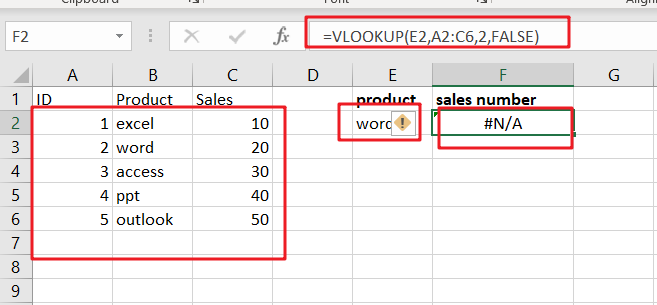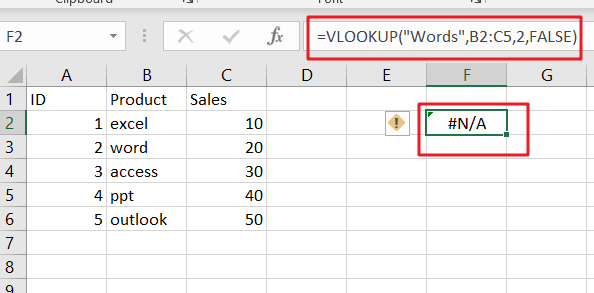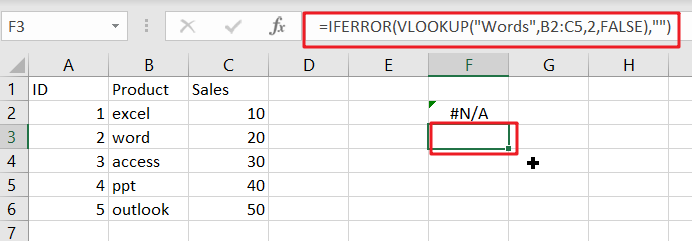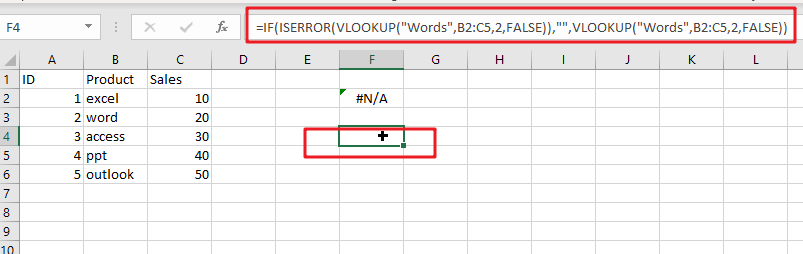# Fix #N/A Error For VLOOKUP From Another Sheet

This post will show you how to fix the #N/A error why it occurs when you extract values from another sheet using VLOOKUP function in Excel 2016,2013,2010 or other Excel versions. How can you correct a #N/A error in VLOOKUP function in Excel. This post will also show you several reasons why VLOOKUP formula is not working and displaying the #N/A, #NAME and #VALUE error messages.

## The Reasons Why VLOOKUP Formula is Not Working

#1 Lookup value is not in the first column of the table array

The VLOOKUP function is that it can only look for values on the left-most column in the table array and if lookup value is not in the first column of the array, and you will see the #N/A error.

Assume that you want to retrieve the number of sales for product “word”, and you can use the following VLOOKUP function:

`=VLOOKUP(E2,A2:C6,2,FALSE)`

You should see that the #N/A error message will be shown, because the lookup value “word” in Cell E2 appears in the second column of the table array argument A2:C6.

To correct the #N/A error, and you need to adjust your VLOOKUP to reference the correct column.#2 #N/A Error in Exact Match VLOOKUP

If you are starting with an exact match and the exact value “Words” is not found, and the #N/A error is returned. Type the following VLOOKUP formula in Cell F2:

`=VLOOKUP("Words",B2:C5,2,FALSE)`#3 #N/A Error in Approximate Match VLOOKUP

If you are starting with an approximate match using VLOOKUP function, and your formula may be return the #N/A error message caused by the following two cases:

If the lookup column is not sorted in ascending order.

If the lookup value is smaller than the smallest value in the lookup array.

## Remove VLOOKUP #N/A Error

When using VLOOKUP function and it can not find any data or when you haven’t entered a lookup value in VLOOKUP formula, and you should get an #N/A error message. And this error message is not helpful at all. You may be want to remove it in your worksheet. And you can use the IFERROR function to remove #N/A error message in VLOOKUP function.

You can put the above VLOOKUP function into the IFERROR function as a new formula, refer to the below formula based on the IFERROR function and VLOOKUP function, it returns a blank string instead of #N/A error.

`=IFERROR(VLOOKUP("Words",B2:C5,2,FALSE),"")`If you are using Excel 2003, and you can use the IF function and the ISERROR function, as the IFERROR function is not available in Excel 2003, type:

`=IF(ISERROR(VLOOKUP("Words",B2:C5,2,FALSE)),"",VLOOKUP("Words",B2:C5,2,FALSE))`

You need to put the VLOOKUP function in there twice to remove #N/A error message in Excel 2003.### Related Functions

• Excel IF function
The Excel IF function perform a logical test to return one value if the condition is TRUE and return another value if the condition is FALSE. The IF function is a build-in function in Microsoft Excel and it is categorized as a Logical Function.The syntax of the IF function is as below:= IF (condition, [true_value], [false_value])….
• Excel ISERROR function
The Excel ISERROR function used to check for any error type that excel generates and it returns TRUE for any error type, and the ISERR function also can be checked for error values except #N/A error, it returns TRUE while the error is #N/A. The syntax of the ISERROR function is as below:= ISERROR (value)….
• Excel IFERROR function
The Excel IFERROR function returns an alternate value you specify if a formula results in an error, or returns the result of the formula.The syntax of the IFERROR function is as below:= IFERROR (value, value_if_error)….
• Excel VLOOKUP function
The Excel VLOOKUP function lookup a value in the first column of the table and return the value in the same row based on index_num position.The syntax of the VLOOKUP function is as below:= VLOOKUP (lookup_value, table_array, column_index_num,[range_lookup])….